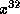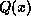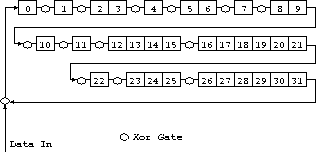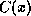Hardware Serial ImplementationNext: Software Implementations Up: Fast CRC32 in Software Previous: Some Examples

# Hardware Serial Implementation

Figure 1 shows a simple shift register implementation of the CRC algorithm. The shift register is initially pre-set to all ones. The data is shifted in bit by bit. As each data bit arrives in the system it is individually multiplied byand added (added is the same as exclusive-or in modulo two arithmetic) to the current remainder. If the remainder then has a term inthen the divisoris subtracted from the current remainder. This proceeds until all the data bits have been presented.Figure 1: CRC32 shift register implementation

If the calculation is being performed in the transmitter then the contents of the shift register are shifted out without further feedback and each bit is added inverted to the tail of the message.

If the calculation is being performed in the receiver then the shift register, will at this point, in the case of an error free transmission, contain the residue.

Richard Black